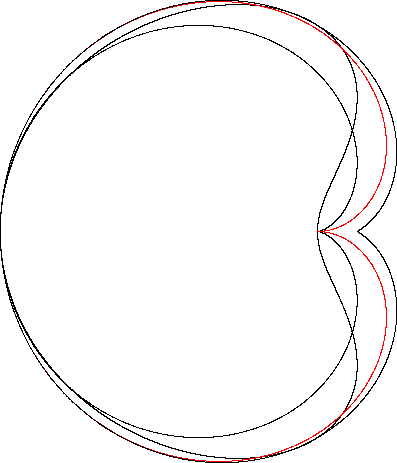Cochleoid
COCHLEOID

Balmoral Software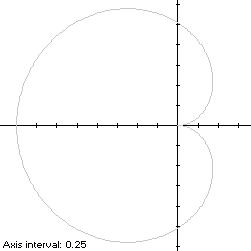A cochleoid having its cusp on the right is a heart-shaped x-symmetric closed curve S with even polar function
r(t) = -2sin(t)/t, t ∈ T,
where t is measured in radians. For a simple curve describing the outer envelope of the cochleoid, T is restricted to the interval [-π,π). The scaling factor -2 is used for comparison with the cardioid.

The curve is traced out in a counterclockwise direction, starting from the cusp at the origin when t = -π. Its minimum abscissa occurs at the limit point (-2,0) as t → 0. Its maximum abscissas occur at the points (0.434467,∓0.541786) when t = ±2.246705, which determine that the cochleoid is non-convex by the multiple local extrema test. The extreme ordinates of the cochleoid occur at the points (-0.621685,∓1.449223) when t = ±1.165561, so the width x height of its bounding rectangle is 2.434467 x 2.898446.

### Metrics

We have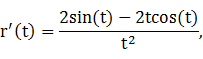so by (L2), the perimeter of this portion of the cochleoid is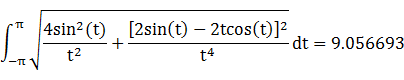By (A2), the corresponding area is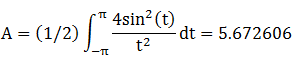By (C2), the centroid abscissa of this portion of the cochleoid is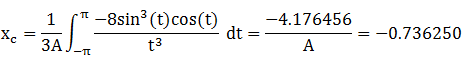The bounding rectangle for inconics is delimited by the cusp at the origin.

### Convex Hull

The convex hull is created by connecting the maximum abscissa points with a vertical line segment of length 1.083572 at x = 0.434467. By (L2), the perimeter of the convex hull is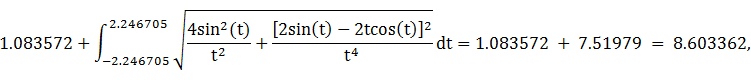which is about 5% shorter than that of the cochleoid.

The line segment of the convex hull creates an isosceles triangle with the origin, having area

(0.434467)(0.541786) = 0.235388
as shown in blue in the left diagram below. The centroid abscissa of this triangle is the average of its vertex abscissas, or 2(0.434467)/3 = 0.289645. By (A2), the remainder of the convex hull has area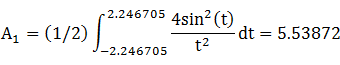By (C2), the associated centroid abscissa is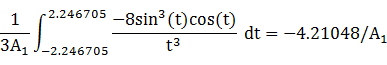The convex hull component metrics can be summarized as follows:
 Area Centroid abscissa Product Region Triangle 0.235388 0.289645 0.068179 Remainder A1 = 5.53872 -4.21048/A1 -4.21048 Total 5.774108 -4.142301
The area of the convex hull is about 2% larger than that of the cochleoid. The centroid abscissa of the convex hull is the weighted average -4.142301/5.774108 = -0.717392.

### Incircle

A candidate incircle is located on the x-axis between the minimum abscissa -2 and the cusp at the origin, and so has radius R = 1 and center abscissa c = -1. For verification, we have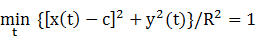### Inellipse

Using z = 0 in Lemma E,
d/dt [x(t) - z]y(t) = d/dt 4sin3(t)cos(t)/t2
has a zero at t* = -0.671886. The corresponding coordinates are
x* = -1.45016

y* = 1.15335

We then have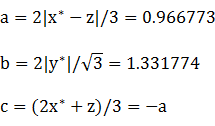For verification,### Circumellipse

Using z = -2 in Lemma E,
d/dt [x(t) - z]y(t) = d/dt [-2sin(t)cos(t)/t + 2]2sin2(t)/t
has a zero at t* = -π/2. The corresponding coordinates are
x* = 0

y* = 4/π

We then have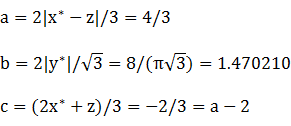For verification,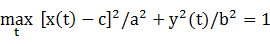### Circumcircle

The radius of a circle centered on the x-axis and circumscribing S is at least its maximum ordinate, so a candidate for the circumcircle has radius R = 1.449223 and center abscissa c = -0.621685. For verification,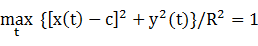### Summary Table

 Perimeter Area Centroid Figure Parameters Incircle R = 1 6.283185 3.141593 (-1,0) Inellipse a = 0.966773b = 1.331774 7.266693 4.044873 (-0.966773,0) Cochleoid Width: 2.434467Height: 2.898446 9.056693 5.672606 (-0.736250,0) Convex hull 8.603362 5.774108 (-0.717392,0) Circumellipse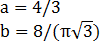8.812839 6.158400 (-0.666667,0) Circumcircle R = 1.449223 9.105737 6.598122 (-0.621685,0)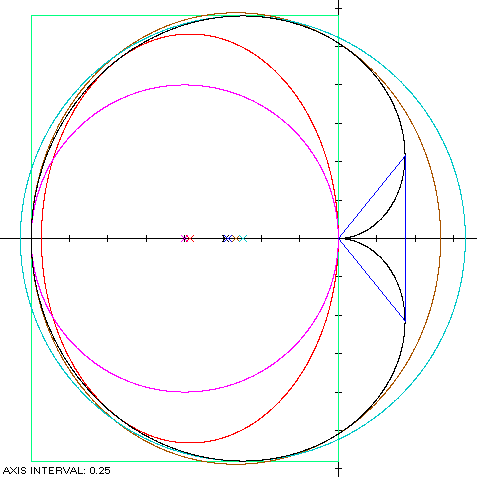The cochleoid (red) is a member of a group of similarly-shaped figures described on these pages, including (inside to outside) the rotated lima bean curve, the cardioid and the Cayley sextic: